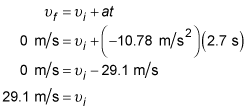##### Physics I: 501 Practice Problems For Dummies (+ Free Online Practice)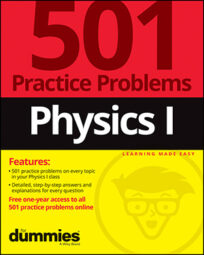In physics, you can calculate how gravity and air resistance affect the vertical velocity of a flying object. For example, if you want a ball to stay in the air for a certain amount of time, you can calculate how fast it has to be moving when you throw it.

Here are some practice questions that you can try.

## Practice questions

1. A bottle rocket fires straight up off the ground, landing 2.8 seconds later at the same spot from which it launched. How long after launch does the rocket reach its highest point, assuming there is no air resistance?

Round your answer to the nearest tenth of a second.

2. Sam tosses a 1.2-kilogram billiard ball straight up into the air, where it is subjected to an air resistance equivalent to 10 percent of its weight. If the ball reaches the apex of its flight after 2.7 seconds, with what speed does Sam toss the billiard ball into the air?

Round your answer to the nearest meter per second.

The following are the answers to the practice questions:

1. 1.4 s

When a projectile launches from and lands at the same vertical position, it reaches its apex (highest point) halfway through the trip — both in terms of horizontal distance traveled and time of flight. Therefore, the rocket reaches its highest point of flight after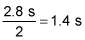2. 29 m/s

Weight is vernacular for force of gravity, so the force of air resistance FF is equal to 0.1FG, where FG is the force of gravity. Draw a free-body diagram: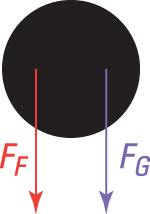The friction force FF (air resistance) points downward because friction always opposes an object's motion, which — on the ball's way to the highest point — is upward. Now use the force form of Newton's second law to calculate the billiard ball's acceleration: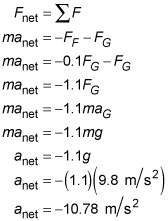The apex (highest point) of a projectile's arc is the place where the projectile's vertical velocity changes from a positive (upward) value to a negative (downward) one. Therefore, its vertical velocity at the top must be 0 meters per second (the only number between positives and negatives). So use the velocity-time formula to solve for the ball's initial velocity at launch, 2.7 seconds prior to it having a "final" velocity of 0 meters per second: